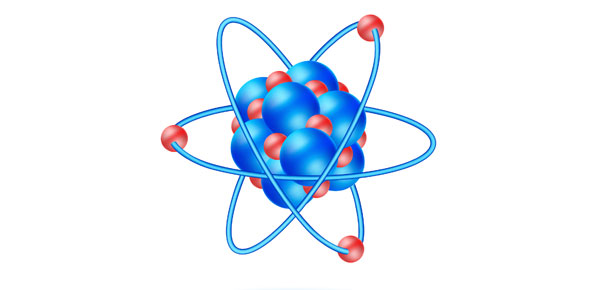# Physical Science-Practice Test For Chapter 13

18 Questions | Total Attempts: 76SettingsCreate your own Quiz• 1.
A wave is a back and motion that transmits________
• 2.
What is the name of the substance that the energy of wave travels through
• A.

Conductor

• B.

Medium

• C.

Anode

• D.

Insulator

• 3.
The name of the back and forth motion of waves
• A.

Rolling

• B.

Charging

• C.

Ocilation

• D.

Induction

• 4.
The types of waves
• A.

Transverse

• B.

Vertical

• C.

Horizontal

• D.

Longitudinal

• 5.
A wave can be described by
• A.

Wavelength

• B.

Color

• C.

Amplitude

• D.

Frequency

• E.

Speed

• F.

Temperature

• 6.
_____________is the distance between two crests
• 7.
__________ is a waves height
• 8.
The number of complete waves that pass a certain point in a second
• 9.
The rate at which at wave travels through a medium
• 10.
In 1960 Robert Boyle proved that sound cannot travel through a________
• A.

Water

• B.

A vacuum

• C.

Glass

• D.

Earth

• 11.
The intensity of sound waves is the_______of the wave
• 12.
The more intense the sound wave the_______we think it is
• 13.
A unit of sound
• A.

Inch

• B.

Pound

• C.

Decibel

• D.

Gallon

• 14.
Audible sound is from________
• A.

5Hz-10Hz

• B.

10,000Hz-100,000Hz

• C.

16Hz-25,000Hz

• D.

16Hz-20,000Hz

• 15.
The speed of sound
• A.

340m/s

• B.

500m/s

• C.

1020m/s

• D.

270m/s

• 16.
Sound waves always bend_______the medium that slows them down
• A.

Towards

• B.

Away

Related TopicsBack to top
×

Wait!
Here's an interesting quiz for you.